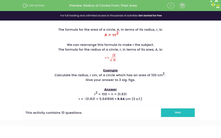# Radius of Circles From Their Area

In this worksheet, students calculate the radius of a circle given its area.Key stage:  KS 3

Curriculum topic:   Geometry and Measures

Curriculum subtopic:   Solve Problems Involving Perimeters and Areas of 2D Shapes

Difficulty level:#### Worksheet Overview

The formula for the area of a circle, A, in terms of its radius, r, is:

A = πr2

We can rearrange this formula to make r the subject.

The formula for the radius of a circle, r, in terms of its area, A, is:Example

Calculate the radius, r cm, of a circle which has an area of 100 cm2